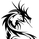1260 vues
request for jangseohee
```//@version=2
study(title='[RS]DeMark Range Expansion Index V0', shorttitle='REI', overlay=false)
//  ||  Tom DeMark's Range Expansion Index
//  ||  AFL Implementation by Tomasz Janeczko
length = input(5)

HighMom = high - high
LowMom = low - low

Cond1 = high >= low or high >= low
Cond2 = high >= close or high >= close
Cond3 = low <= high or low <= high
Cond4 = low <= close or low <= close

Cond = ( Cond1 or Cond2 ) and ( Cond3 or Cond4 )

Num = Cond ? HighMom + LowMom : 0
Den = abs(HighMom) + abs(LowMom)

TDREI = 100 * sum( Num, length )/sum( Den, length )

plot(title='REI', series=TDREI, color=black)
hline(title='OB', price=80, color=black)
hline(title='OS', price=-80, color=black)```

## CommentairesIt's lack some conditions. The original have 12 conditions. : )
I've gone crazy when I saw it.
Répondre